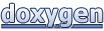# Assimp: aiQuaterniont< TReal > Class Template Reference

#### assimp - Open Asset Import Library

 Assimp  v3.1.1 (June 2014)
aiQuaterniont< TReal > Class Template Reference

Represents a quaternion in a 4D vector. More...

## Public Member Functions

aiQuaterniont ()

aiQuaterniont (TReal pw, TReal px, TReal py, TReal pz)

aiQuaterniont (const aiMatrix3x3t< TReal > &pRotMatrix)
Construct from rotation matrix. More...

aiQuaterniont (TReal rotx, TReal roty, TReal rotz)
Construct from euler angles. More...

aiQuaterniont (aiVector3t< TReal > axis, TReal angle)
Construct from an axis-angle pair. More...

aiQuaterniont (aiVector3t< TReal > normalized)
Construct from a normalized quaternion stored in a vec3. More...

aiQuaterniontConjugate ()
Compute quaternion conjugate. More...

bool Equal (const aiQuaterniont &o, TReal epsilon=1e-6) const

aiMatrix3x3t< TReal > GetMatrix () const
Returns a matrix representation of the quaternion. More...

aiQuaterniontNormalize ()
Normalize the quaternion. More...

bool operator!= (const aiQuaterniont &o) const

aiQuaterniont operator* (const aiQuaterniont &two) const
Multiply two quaternions. More...

bool operator== (const aiQuaterniont &o) const

aiVector3t< TReal > Rotate (const aiVector3t< TReal > &in)
Rotate a point by this quaternion. More...

## Static Public Member Functions

static void Interpolate (aiQuaterniont &pOut, const aiQuaterniont &pStart, const aiQuaterniont &pEnd, TReal pFactor)
Performs a spherical interpolation between two quaternions and writes the result into the third. More...

## Public Attributes

TReal w
w,x,y,z components of the quaternion More...

TReal x

TReal y

TReal z

## Detailed Description

### template<typename TReal> class aiQuaterniont< TReal >

Represents a quaternion in a 4D vector.

## Constructor & Destructor Documentation

template<typename TReal >
 aiQuaterniont< TReal >::aiQuaterniont ( )
inline
template<typename TReal >
 aiQuaterniont< TReal >::aiQuaterniont ( TReal pw, TReal px, TReal py, TReal pz )
inline
template<typename TReal >
 aiQuaterniont< TReal >::aiQuaterniont ( const aiMatrix3x3t< TReal > & pRotMatrix )
inlineexplicit

Construct from rotation matrix.

Result is undefined if the matrix is not orthonormal.

template<typename TReal >
 aiQuaterniont< TReal >::aiQuaterniont ( TReal rotx, TReal roty, TReal rotz )
inline

Construct from euler angles.

template<typename TReal >
 aiQuaterniont< TReal >::aiQuaterniont ( aiVector3t< TReal > axis, TReal angle )
inline

Construct from an axis-angle pair.

template<typename TReal >
 aiQuaterniont< TReal >::aiQuaterniont ( aiVector3t< TReal > normalized )
inlineexplicit

Construct from a normalized quaternion stored in a vec3.

## Member Function Documentation

template<typename TReal >
 aiQuaterniont< TReal > & aiQuaterniont< TReal >::Conjugate ( )
inline

Compute quaternion conjugate.

template<typename TReal >
 bool aiQuaterniont< TReal >::Equal ( const aiQuaterniont< TReal > & o, TReal epsilon = `1e-6` ) const
inline
template<typename TReal >
 aiMatrix3x3t< TReal > aiQuaterniont< TReal >::GetMatrix ( ) const
inline

Returns a matrix representation of the quaternion.

template<typename TReal >
 void aiQuaterniont< TReal >::Interpolate ( aiQuaterniont< TReal > & pOut, const aiQuaterniont< TReal > & pStart, const aiQuaterniont< TReal > & pEnd, TReal pFactor )
inlinestatic

Performs a spherical interpolation between two quaternions and writes the result into the third.

Parameters
 pOut Target object to received the interpolated rotation. pStart Start rotation of the interpolation at factor == 0. pEnd End rotation, factor == 1. pFactor Interpolation factor between 0 and 1. Values outside of this range yield undefined results.
template<typename TReal >
 aiQuaterniont< TReal > & aiQuaterniont< TReal >::Normalize ( )
inline

Normalize the quaternion.

template<typename TReal >
 bool aiQuaterniont< TReal >::operator!= ( const aiQuaterniont< TReal > & o ) const
template<typename TReal >
 aiQuaterniont< TReal > aiQuaterniont< TReal >::operator* ( const aiQuaterniont< TReal > & two ) const
inline

Multiply two quaternions.

template<typename TReal >
 bool aiQuaterniont< TReal >::operator== ( const aiQuaterniont< TReal > & o ) const
template<typename TReal >
 aiVector3t< TReal > aiQuaterniont< TReal >::Rotate ( const aiVector3t< TReal > & in )
inline

Rotate a point by this quaternion.

## Member Data Documentation

template<typename TReal >
 TReal aiQuaterniont< TReal >::w

w,x,y,z components of the quaternion

template<typename TReal >
 TReal aiQuaterniont< TReal >::x
template<typename TReal >
 TReal aiQuaterniont< TReal >::y
template<typename TReal >
 TReal aiQuaterniont< TReal >::z
The documentation for this class was generated from the following files:
Generated on Sun Feb 21 2016 19:42:29 for Assimp by1.8.11